New update is available. Click here to update.
Topics

# Ninja and Tree

Ninja0/200
0 upvote## Problem statement

You are given an undirected tree with ‘N’ vertices in the form of an array of ‘EDGES’ and ‘Q’ queries in ‘QUERIES’ array and root in the vertex ‘1’. You have also been given array ‘COLOR’ where ‘COLOR[i]’ represents the initial color of i-th vertex.

‘QUERIES’ is a 2D array where each row ‘QUERIES[i]’ represents a query of type ‘QUERIES[i]’.

You should process the queries of the two types:

1) Change the colours of all vertices in the subtree of the vertex ‘QUERIES[i]’ to the colour ‘QUERIES[i]’.

2) Find the number of different colours in the subtree of the vertex ‘QUERIES[i]’.

For Example:
``````Let ‘N’ = 5, ‘EDGES’ = [[1, 2], [1, 3], [2, 4], [2, 5]], ‘QUERIES’ = [[1, 2, 3], [2, 1], [1, 3, 2], [2, 1]] and ‘COLOR’ = [1, 1, 1, 1, 1].
The initial tree will be:
``````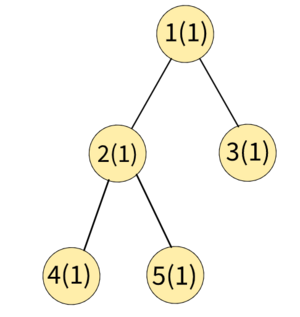``````After processing the query [1, 2, 3] i.e. colour all vertices in the subtree of 2 to 3.
``````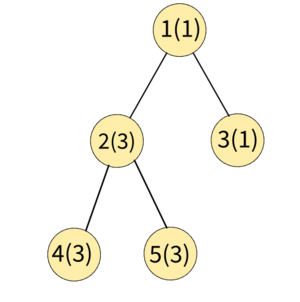``````Now the number of different colours in subtree of the vertex 1 is 2.
After processing the query [1, 3, 2] i.e. colour all vertices in the subtree of 3 to 2.
``````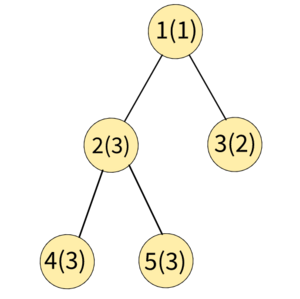``````Now the number of different colours in subtree of the vertex 1 are 3.
Hence the answer to this test case is 2 and 3 (print on separate lines).
``````
Detailed explanation ( Input/output format, Notes, Images )
Constraints :
``````1 ≤ T ≤ 10
1 ≤ N ≤ 1000
1 ≤ Q ≤ 1000
1 ≤ COLOR[i] ≤ 60

Time limit: 1 sec
``````
Sample Input 1 :
``````2
5 4
1 1 1 1 1
1 2
1 3
2 4
2 5
1 2 3
2 1
1 3 2
2 1
3 2
1 1 1
1 2
1 3
1 2 2
2 1
``````
Sample Output 1 :
``````2
3
2
``````
Explanation of Sample Output 1 :
``````Test Case 1:
The initial tree will be:
````````````After processing the query [1, 2, 3] i.e. color all vertices in subtree of 2 to 3.
````````````Now the number of different colors in subtree of the vertex 1 are 2.
After processing the query [1, 3, 2] i.e. color all vertices in subtree of 3 to 2.
````````````Now the number of different colors in subtree of the vertex 1 are 3.
Hence the answer to this test case is 2 and 3 (print on separate lines).

Test Case 2:
The initial tree will be:
``````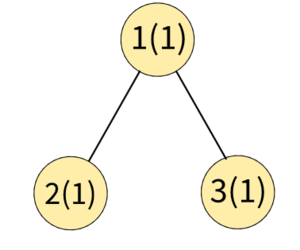``````After processing the query [1, 2, 2] i.e. color all vertices in subtree of 2 to 2.
``````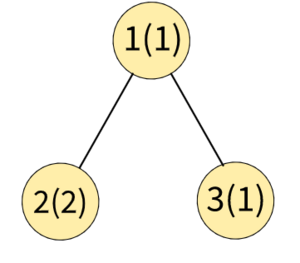``````Now the number of different colors in the subtree of the vertex 1 are 2.
Hence the answer to this test case is 2.
``````
Sample Input 2 :
``````2
2 2
1 3
1 2
1 1 2
2 1
1 1
1
2 1
``````
Sample Output 2 :
``````1
1
``````
Explanation of Sample Output 2 :
``````Test Case 1:
The initial tree will be:
``````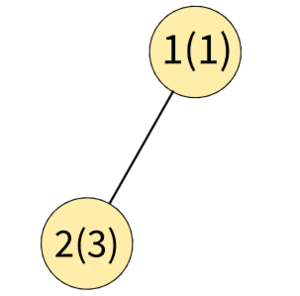``````After processing the query [1, 1, 2] i.e. color all vertices in the subtree of 1 to 2.
``````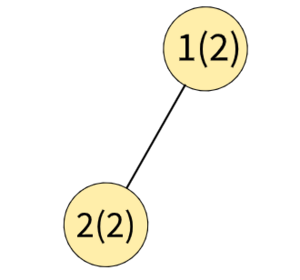``````Now the number of different colors in subtree of vertex 1 is 1. Hence answer to this testcase will be 1.

Test Case 2:
Initially, tree will be:
````````````The number of different colors in the subtree of vertex 1 is only 1. Hence answer to this testcase will be 1.
``````Console